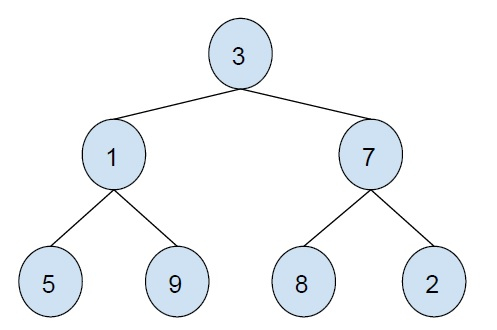# Zig Zag Level order traversal of a tree using single queue in C++

In this problem, we are given a binary tree. Our task is to print the zigzag level order traversal of the tree. For this traversal, we will use a single queue only.

Let’s take an example to understand the problem,Output

3    1    7    2    8    9    5

To solve this problem using a single queue, we will sue an extra separation flag along with the queue and direction flag.

Now, we will traverse the tree level by level, insert root element, now insert for every element of the queue insert its child node to the queue. If NULL is encountered, we will check the direction and then traverse the elements in the direction given. Then we will keep on inserting until we visit the last NULL.

## Example

Program to illustrate our solution,

Live Demo

#include <bits/stdc++.h>
using namespace std;
struct Node {
int data;
struct Node *left, *right;
};
struct Node* insertNode(int data) {
struct Node* node = new struct Node;
node->data = data;
node->left = node->right = NULL;
return (node);
}
void zigZagTraversal(struct Node* root, int n){
struct Node* queue[2 * n];
int top = -1;
int front = 1;
queue[++top] = NULL;
queue[++top] = root;
queue[++top] = NULL;
int prevFront = 0, count = 1;
while (1) {
struct Node* curr = queue[front];
if (curr == NULL) {
if (front == top)
break;
else {
if (count % 2 == 0) {
for (int i = prevFront + 1; i < front; i++)
cout<<queue[i]->data<<"\t";
}
else {
for (int i = front - 1; i > prevFront; i--)
cout<<queue[i]->data<<"\t";
}
prevFront = front;
count++;
front++;
queue[++top] = NULL;
continue;
}
}
if (curr->left != NULL)
queue[++top] = curr->left;
if (curr->right != NULL)
queue[++top] = curr->right;
front++;
}
if (count % 2 == 0) {
for (int i = prevFront + 1; i < top; i++)
cout<<queue[i]->data<<"\t";
}
else {
for (int i = top - 1; i > prevFront; i--)
cout<<queue[i]->data<<"\t";
}
}
int main() {
struct Node* root = insertNode(3);
root->left = insertNode(1);
root->right = insertNode(7);
root->left->left = insertNode(5);
root->left->right = insertNode(9);
root->right->left = insertNode(8);
root->right->right = insertNode(2);
cout<<"Zig Zag traversal of the tree is :\n";
zigZagTraversal(root, 7);
return 0;
}

## Output

Zig Zag traversal of the tree is :
3    1    7    2    8    9    5